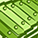# QlikView App Dev

Discussion Board for collaboration related to QlikView App Development.

cancel
Showing results for
Did you mean:Contributor III

## Total value showing wrong in pivot table

Hi,

I am calculating sum of sales value, the total showing in the pivot table is different from the total in excel. Sales values are both positive and negative.

For eg; the total i got from pivot table is 35971.85 but the same calculated in excel showing 36033.07.

10 RepliesChampion

Hi Remya,

Could you attach a sample file?Creator III

it may be because of your Dimension, there may be some null values in the data.

Go to Dimension tab-> then uncheck supress when value is nullContributor III
Author

Hi,Champion

Try like this as your expression,

Sum(Aggr(Sum(Sales), Region, RouteCode))Contributor III
Author

Actually this is in a self service report, so the dimension will change.

This is the expression am using for sales value

Sum({\$<DateKey={">=\$(=Date(vFromDate,'YYYYMMDD'))<=\$(=Date(vToDate,'YYYYMMDD'))"}, Year=, Quarter=, Month=, WeekName=, Date=>} \$(eSales))

-

Sum({\$<DateKey={">=\$(=Date(vFromDate,'YYYYMMDD'))<=\$(=Date(vToDate,'YYYYMMDD'))"}, Year=, Quarter=, Month=, WeekName=, Date=>} \$(eRent))Champion
 Remya Sujith wrote: Actually this is in a self service report, so the dimension will change.

Is that mean cyclic group or variables? Is it possible to attach your qvw file here?

Preparing examples for Upload - Reduction and Data ScramblingContributor III
Author

Sorry I cannot attach.

It is like the dimension can be chosen by the user, they can select route and sales value or item and sales value or customer and sales value for a range of from date and to date.Champion
 Remya Sujith wrote: It is like the dimension can be chosen by the user, they can select route and sales value or item and sales value or customer and sales value for a range of from date and to date.

Sorry. I just want to know how the dimensions are defined. So that we can include the same in Aggr function.Contributor III
Author

Some of the sub totals are wrong, and the total is calculating by adding those subtotals. If I calculate with values it will give the correct values.